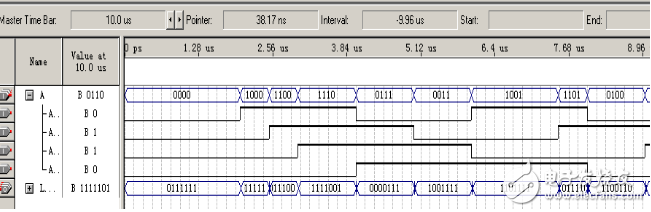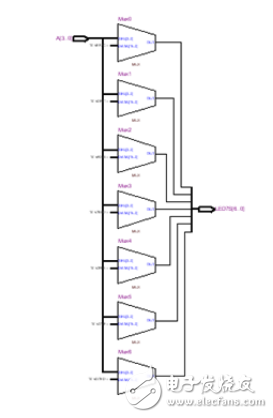﻿ 7段数码管显示的VHDL设计（两款设计方案） - 电子发烧友网

# 7段数码管显示的VHDL设计（两款设计方案）

2018年01月29日 15:48 电子发烧友整理 作者： 用户评论（0

## 7段数码管显示的VHDL设计一：7段数码显示译码器

LIBRARY IEEE;

USE IEEE.STD_LOGIC_1164.ALL; ENTITY DecL7S IS

PORT（A ：IN STD_LOGIC_VECTOR（3 DOWNTO 0）;

LED7S:OUT STD_LOGIC_VECTOR（6 DOWNTO 0） ）; END;

ARCHITECTURE one OF DecL7S IS BEGIN

PROCESS（A） BEGIN

CASE A（3 DOWNTO 0） IS

WHEN “0000”=》LED7S《=”0111111”;

WHEN “0001”=》LED7S《=”0000110”;

WHEN “0010”=》LED7S《=”1011011”;

WHEN “0011”=》LED7S《=”1001111”;

WHEN “0100”=》LED7S《=”1100110”;

WHEN “0101”=》LED7S《=”1101101”;

WHEN “0110”=》LED7S《=”1111101”;

WHEN “0111”=》LED7S《=”0000111”;

WHEN “1000”=》LED7S《=”1111111”;

WHEN “1001”=》LED7S《=”1101111”;

WHEN “1010”=》LED7S《=”1110111”;

WHEN “1011”=》LED7S《=”1111100”;

WHEN “1100”=》LED7S《=”0111001”;

WHEN “1101”=》LED7S《=”1011110”;

WHEN “1110”=》LED7S《=”1111001”;

WHEN “1111”=》LED7S《=”1110001”;

WHEN OTHERS=》 NULL;

END CASE; END PROCESS;

END;

### 波形仿真## 7段数码管显示译码器的VHDL设计二：基于vhdl 实现的4位七段数码管显示

LIBRARY IEEE;

USE IEEE.STD_LOGIC_1164.ALL;

USE ieee.std_logic_unsigned.all;

ENTITY counter0000_ffff IS PORT （ clk， en ：in STD_LOGIC;

set_ch:in std_logic_vector（ 1 downto 0）;

set_num ：in std_logic_vector（ 3 downto 0）;

data ： out STD_LOGIC_VECTOR（6 DOWNTO 0）;

sel ： out STD_LOGIC_VECTOR（2 DOWNTO 0） ）;

END counter0000_ffff;

architecture behave of counter0000_ffff is

signal num，num1，num2，num3，num4： std_logic_vector （3 downto 0）;

signal num_counter:std_logic;

begin

a:process（ clk ）

variable cc： integer range 0 to 3：=0;

begin

if（ clk = ‘0’ ） then

case cc is

when 0 =》 num 《= num2;sel 《= “011”;cc：=1;

when 1 =》 num 《= num3;sel 《= “010”;cc：=2;

when 2 =》 num 《= num4;sel 《= “001”;cc：=3;

when 3 =》 num 《= num1;sel 《= “000”;cc：=0;

end case;

case num is

when “0000” =》 data 《= “0111111”;

when “0001” =》 data 《= “0000110”;

when “0010” =》 data 《= “1011011”;

when “0011” =》 data 《= “1001111”;

when “0100” =》 data 《= “1100110”;

when “0101” =》 data 《= “1101101”;

when “0110” =》 data 《= “1111101”;

when “0111” =》 data 《= “0000111”;

when “1000” =》 data 《= “1111111”;

when “1001” =》 data 《= “1101111”;

when “1010” =》 data 《= “1110111”;

when “1011” =》 data 《= “1111100”;

when “1100” =》 data 《= “0111001”;

when “1101” =》 data 《= “1011110”;

when “1110” =》 data 《= “1111001”;

when “1111” =》 data 《= “1110001”;

end case;

end if;

end process;

b:process（ clk ） –自加一

begin

if（ clk = ‘0’） then

if（ en = ‘1’） then

CASE set_ch IS

when “00” =》 num1《=set_num;

when “01” =》 num2《=set_num;

when “10” =》 num3《=set_num;

when “11” =》 num4《=set_num;

end case; else if num_counter = ‘0’ then

if num1 = “1111” then num1 《= “0000”;

num2 《= num2 + 1;

else num1 《= num1 +1;

end if;

if num2 = “1111” then num2 《= “0000”;

num3 《= num3 + 1;

end if;

if num3 = “1111” then

num3 《= “0000”;

num4 《= num4 + 1;

end if;

if num4 = “1111” then

num4 《= “0000”;

end if;

end if;

END IF;

end if;

end process;

c:process（ clk ） –分频

variable a:integer range 0 to 9999：=0;

begin if（ clk = ‘1’） then

if（ a = 9999 ） then

num_counter 《= not num_counter;

a ：= 0;

else a ：= a + 1;

end if;

end if;

end process;

end behave;

(0) 0%

(0) 0%

( 发表人：李建兵 )

## 用户评论

### 发表评论

用户评论
评价:好评中评差评

发表评论，获取积分！ 请遵守相关规定！

﻿